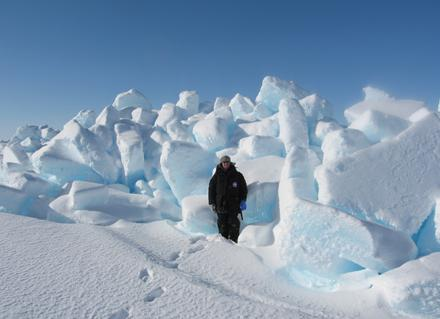Search IntMath
Close

# The melting Arctic - a disturbing application of math

By Murray Bourne, 20 Mar 2008

The article Maths and climate change: the melting Arctic is from the Millennium Mathematics Project people.

It features the work of Peter Wadhams, Professor of Ocean Physics and Head of the Polar Ocean Physics Group at University of Cambridge.

Wadhams outlines the seriousness of an Arctic meltdown:

"As the ice area decreases you're replacing an area of white, which reflects about 90% of the solar radiation, by open water, which reflects less than 10%. As the sea ice retreats you're absorbing a lot more radiation and this increases the rate of warming at high latitudes." This effect can raise the global average rate of warming by 20%.Image source.

The article goes on to describe a simple ice growth model that involves differentiation (from calculus) and vectors. The model predicts that the growth of ice (thickness, h) over time is proportional to the square root of the elapsed time:

h(t) = kt (where k is a constant).

This means that when ice is thin, it grows quickly, but as time goes on the rate of growth decreases.

Then Wadham goes on to model the movement of ice that is floating on water and is being acted on by the wind and by the Coriolis force.

This is mathematics that I wish we didn't have to concern ourselves so much with. But we do...

Check out the full Plus Magazine article.

[Thanks to Li-sa, one of my loyal readers, for the link to Plus Magazine.]

### Comment Preview

HTML: You can use simple tags like <b>, <a href="...">, etc.

To enter math, you can can either:

1. Use simple calculator-like input in the following format (surround your math in backticks, or qq on tablet or phone):
a^2 = sqrt(b^2 + c^2)
(See more on ASCIIMath syntax); or
2. Use simple LaTeX in the following format. Surround your math with $$ and $$.
$$\int g dx = \sqrt{\frac{a}{b}}$$
(This is standard simple LaTeX.)

NOTE: You can mix both types of math entry in your comment.